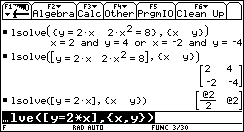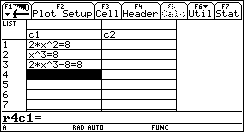## Texas Instruments TI-89 and TI-92 Plus Computer

Last page update: January 03, 2000
 Multiple Equation Solver - for the TI-89 and TI-92 Plus Version 1.1   (January 03, 2000) Download [lsolve.zip] Description Solves a system of linear or non-linear equations in a list or matrix. Screen shotsFigure 1.Figure 2. Documentation Usage: lSolve(Expr,Var)  Expr: A single equation or a system of equations in a list or matrix.  Var: The variable or list of variables to solve for.  For a system of equations is is simplest to create a list in the Data/Matrix Editor (Figure 2.) and use that MAT or LIST variable as the argument to lSolve() (Figure 1. command line). Multiple solutions to systems with multiple variables will be returned in a matrix if Expr is a matrix. This feature was implemented so that a large solution can be more easily viewed as a matrix and so that the matrix can be used as a list of vectors. The returned value can also be stored in a variable (i.e., lSolve(ExpList,VarList)->m) so that you can view it in the Data/Matrix Editor.  Return value: The solutions to the equation or system of equations. If Var is a single variable or if Expr is a list, an expression is returned. If Expr is a matrix, a matrix is returned.  Examples: 1. lSolve({2x=4,x2=4},x)     x = 2  2. lSolve({y=2x,2x2=8},{x,y})     {x =2 or x=-2,y=4 or y=-4} 3. lSolve([y=2x,2x2=8],{x,y})     [[ 2,  4]      [-2, -4]]